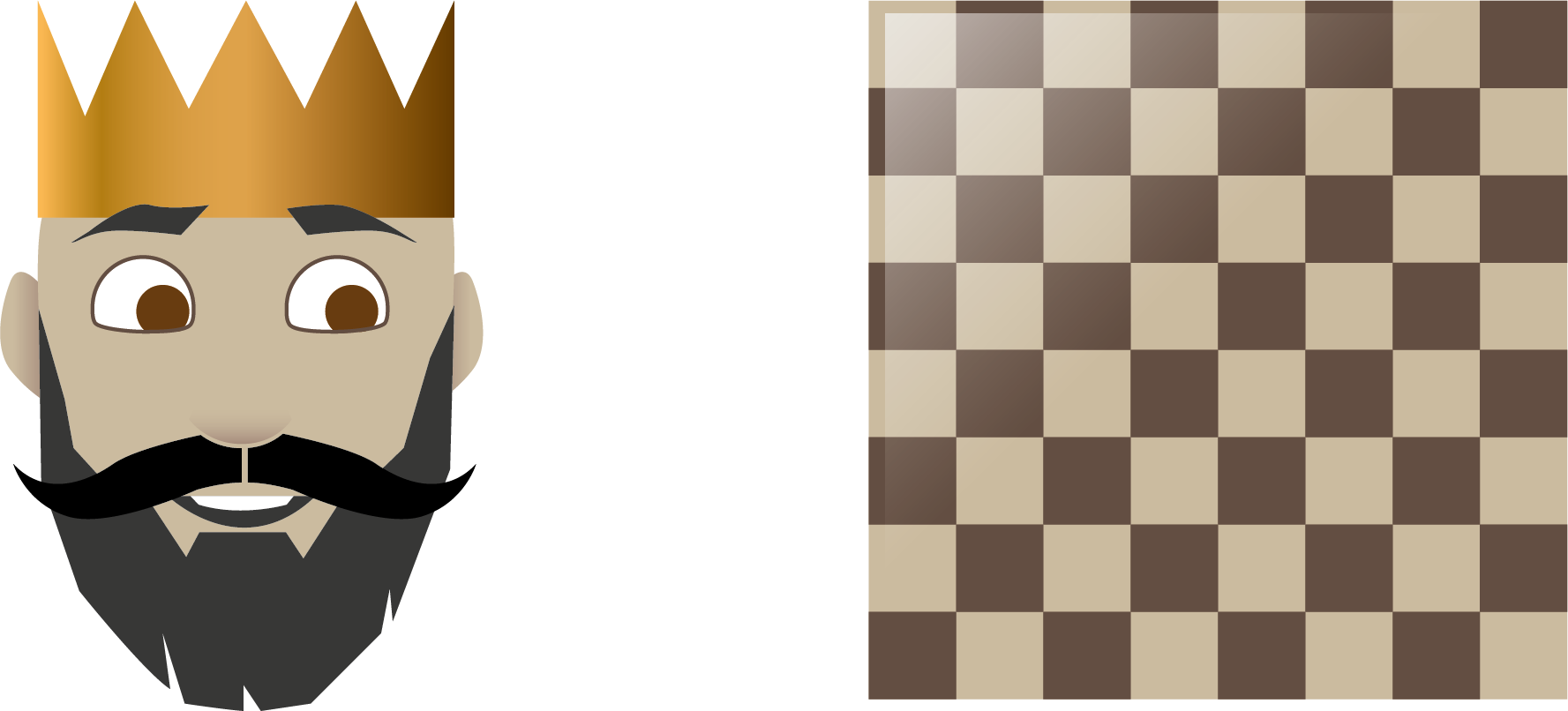Math Topics

# What Are Powers in Math?

Video Crash Courses

Want to watch animated videos and solve interactive exercises about powers?

When you need to express a product consisting of repeating The trick of moving the decimal point when dividing by 10, is similar to a trick you can use when factors, you would rather use powers (${a}^{n}$). If you try to calculate this product, the answer tends to become very large or very small. Using powers is the method that lets you skip these big calculations. In short, we use powers to handle multiplications and numbers that are very large or very small. But what exactly are powers?

The base is the number you want to multiply by itself. The exponent tells you how many times you need to multiply the base by itself. I imagine that the base is on the ground floor and the exponent is in the attic. In short, a power is an expression that is made up of a base and an exponent. Let’s go more in depth and see what this means:

Rule

### Powers

$a$ is the base and $n$ is the exponent.

Example 1

What does ${3}^{4}$ mean?

The formula says that $3$ is the base and that $4$ is the exponent. The exponent tells us that $3$ needs to be multiplied by itself $4$ times:

What is ${2}^{7}$? From the formula we can see that $2$ is the base and that the exponent is $7$. This means that $2$ needs to be multiplied by itself 7 times, which gives you

A rich, Indian king wanted someone to come up with something he could do. One day, Sissa ben Dahir brought a game before the king. The game consisted of a board with $8$ times $8$ tiles, which adds up to $64$ tiles in total, and some game pieces supposed to represent people at court.

The king was very excited, and wanted to reward Sissa. Sissa said he wanted $1$ grain of rice for the first tile, $2$ for the second, $4$ for the third, and so on all the way to the final tile on the board. He wanted the number of rice grains to double for every tile. The king thought this was a very modest wish and granted it without second thought. But was this really a good deal for the king? How many grains of rice would Sissa get for the 8. tile, and how many would he get for the 64. tile?

For the first tile he got $1$ grain of rice. For the second tile he got $2$ grains of rice. For the third tile he got $2\cdot 2={2}^{2}=4$ grains of rice. Tile number four got him $2\cdot 2\cdot 2={2}^{3}=8$ grains of rice. From the pattern you can see that for each tile, the exponent is one less than the number of the tile. Therefore tile number eight gets Sissa ${2}^{7}=128$ grains of rice. To find out how many he gets from tile number sixty four you have to calculate ${2}^{63}$. That is the same as multiplying $2$ with itself $63$ times,

 ${2}^{63}=9\phantom{\rule{0.17em}{0ex}}223\phantom{\rule{0.17em}{0ex}}372\phantom{\rule{0.17em}{0ex}}036\phantom{\rule{0.17em}{0ex}}854\phantom{\rule{0.17em}{0ex}}775\phantom{\rule{0.17em}{0ex}}808,$

which is about 9 quintillion grains of rice!